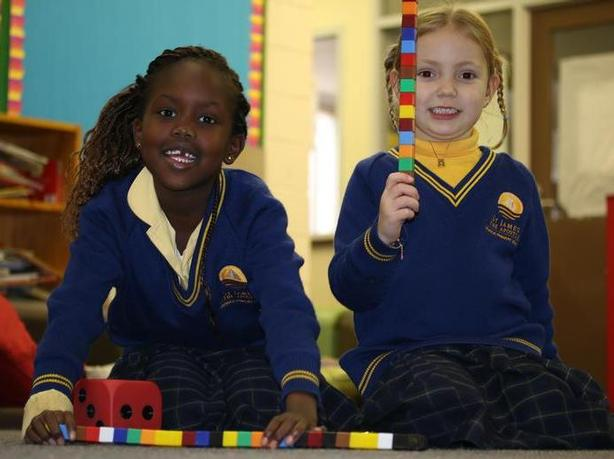# Mathematics

Mathematics is an important human endeavour, which includes study about Number and Algebra, Measurement and Geometry, and Statistics and Probability.

The Mathematics program at St James the Apostle Primary School has its structure based on the Contemporary Teaching and Learning of Mathematics (CTLM) program developed by Catholic Education Melbourne and the Australian Catholic University.At St James the Apostle Primary School we adopt teaching and learning strategies in Mathematics that enable children to be actively engaged in the learning process, learning mathematics concepts and skills that can be used in real life.

Students use a range of materials and move towards deep understanding and abstract thinking of mathematics and at all levels, students are encouraged to share their strategies and reflect on their mathematical thinking.

Students are provided with rich mathematical learning experiences that allow them to participate in oral activities, written activities, manipulation of concrete materials, games, problem solving, open-ended tasks, problem-solving task centres and the use of calculators and computer applications to develop mathematical knowledge and understanding.

Mathematics learning and teaching involves developing skills in the following areas:

• Number and Algebra
• Measurement and Geometry
• Statistics and Probability

Students work towards becoming proficient mathematicians through being learners who:

• Understand;
• Are fluent;
• Can solve problems; and
• Give reasons.

Rich mathematical learning experiences:

• Engage the learner and make explicit the links between mathematics and the real world;
• Are targeted to the needs of the students;
• Develop a knowledge of facts and mathematical processes;
• Develop a deep understanding of how mathematics is used and applied;
• Encourage problem solving and risk taking;
• Provide the opportunity for students and teachers to communicate using mathematical language;
• Develop estimation skills; and
• Provide opportunities to articulate strategies and reflect on learning.